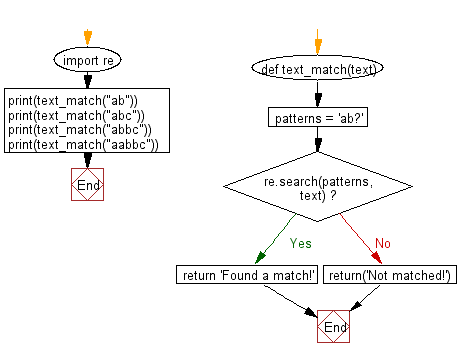﻿ Python Exercises: Matches a string that has an a followed by zero or one 'b' - w3resource# Python: Matches a string that has an a followed by zero or one 'b'

## Python Regular Expression: Exercise-4 with Solution

Write a Python program that matches a string that has an a followed by zero or one 'b'.

Sample Solution:-

Python Code:

``````import re
def text_match(text):
patterns = 'ab?'
if re.search(patterns,  text):
return 'Found a match!'
else:
return('Not matched!')

print(text_match("ab"))
print(text_match("abc"))
print(text_match("abbc"))
print(text_match("aabbc"))
```
```

Sample Output:

```Found a match!
Found a match!
Found a match!
Found a match!
```

Flowchart:## Visualize Python code execution:

The following tool visualize what the computer is doing step-by-step as it executes the said program:

Python Code Editor:

Have another way to solve this solution? Contribute your code (and comments) through Disqus.

What is the difficulty level of this exercise?

﻿